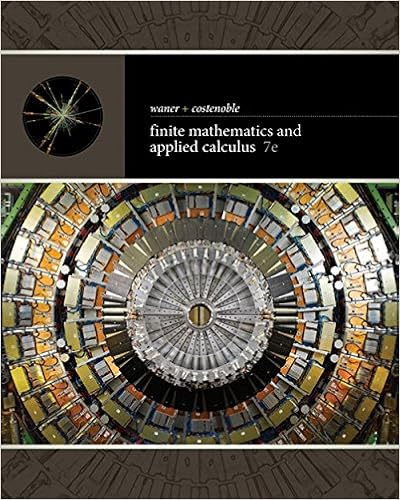# ec201 tutorial wk 11 - Tutorial Exercise Tutorial PartA...

• Test Prep
• ShaniyaKhan7
• 3

This preview shows page 1 - 2 out of 3 pages.

##### We have textbook solutions for you!
The document you are viewing contains questions related to this textbook.The document you are viewing contains questions related to this textbook.
Chapter 3 / Exercise 54
Finite Mathematics and Applied Calculus
Costenoble/WanerExpert Verified
Tutorial Exercise Tutorial PartA – Theory of the IS-LM ModelConceptual Problems: Txt bk pp.265IS relationship/curve:1. What is the IS curve? How does the IS curve relate to the model of aggregate demand developed in Chapter 8?2. a. Explain in words how and why the multiplier αG, and the interest sensitivity of Investment (parameter ‘b’ in [I = Io – bi] affect the slope of the IS curve.b. Explain why the slope of the IS curve is a factor in determining the workings of monetary policy. 3. What causes the IS curve to shift?LM relationship/curve:1. What is the LM curve? How does the LM curve relate to the model of the money market developed in Chapter 14 and 15?2. a. Explain in words how and why the income and interest sensitivity of the demand for real balances (parameter ‘k’ and parameter ‘h’ in [L= kY - hi] affect the slope of the LM curve.b. Explain why the slope of the LM curve is a factor in determining the workings of fiscal policy.3. What causes the LM curve to shift?
##### We have textbook solutions for you!
The document you are viewing contains questions related to this textbook.The document you are viewing contains questions related to this textbook.
Chapter 3 / Exercise 54
Finite Mathematics and Applied Calculus
Costenoble/WanerExpert Verified
•••